Journal of Integer Sequences, Vol. 13 (2010), Article 10.1.3

## Sets with Even Partition Functions and 2-adic Integers II

### N. Baccar Université de Sousse ISITCOM Hammam Sousse Dép. de Math Inf. 5 Bis Rue 1 Juin 1955 4011 Hammam Sousse Tunisie A. Zekraoui Université de Monastir F. S. M. Dép. de Math. Avenue de l'environnement 5000 Monastir Tunisie

Abstract:

For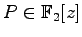with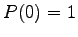and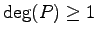, let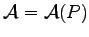be the unique subset of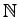such that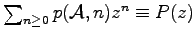(mod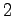), where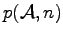is the number of partitions of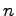with parts in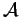. Let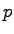be an odd prime number, and let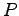be irreducible of order; i.e.,is the smallest positive integer such thatdivides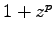in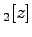. N. Baccar proved that the elements of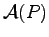of the form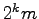, where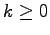and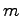is odd, are given by the-adic expansion of a zero of some polynomial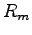with integer coefficients. Let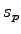be the order ofmodulo, i.e., the smallest positive integer such that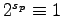(mod). Improving on the method with whichwas obtained explicitly only when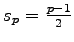, here we make explicitwhen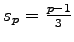. For that, we have used the number of points of the elliptic curve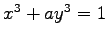modulo.

Full version:  pdf,    dvi,    ps,    latex

Received July 16 2009; revised version received December 23 2009. Published in Journal of Integer Sequences, December 31 2009.

Return to Journal of Integer Sequences home page